# Constructing, measuring and using geometric notation

### Term 3 starting in week 1 :: Estimated time: 3 weeks

• Understand and use letter and labelling conventions including those for geometric figures
• Draw and measure line segments including geometric figures
• Understand angles as a measure of turn
• Classify angles
• Measure angles up to 1800
• Draw angles up to 1800
• Draw and measure angles between 1800 and 3600
• Identify perpendicular and parallel lines
• Recognise types of triangle
• Identify polygons up to a decagon
• Construct triangles using SSS
• Construct triangles using SSS, SAS and ASA
• Construct more complex polygons
• Interpret simple pie charts using proportion
• Interpret pie charts using a protractor
• Draw pie charts

This page should remember your ticks from one visit to the next for a period of time. It does this by using Local Storage so the information is saved only on the computer you are working on right now.

## Lesson Starters

Here are some suggestions for whole-class, projectable resources which can be used at the beginnings of each lesson in this block.

### 1st Lesson#### Angle Estimates

Estimate the sizes of each of the angles then add your estimates together.

### 2nd Lesson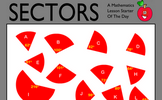#### Sectors

Work out which sectors fit together to make complete circles. Knowledge of the sum of the angles at a point will help find more than one correct solution to this puzzle.

### 3rd Lesson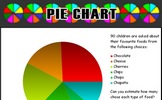#### Pie Chart

An exercise in estimating what the sectors of a pie chart represent.

### 4th Lesson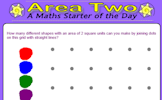#### Area Two

How many different shapes with an area of 2 square units can you make by joining dots on this grid with straight lines?

### 5th Lesson#### How Many Triangles? 1

How many Triangles can you find in the diagram?

### 6th Lesson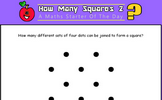#### How Many Squares? 2

How many squares can be found by joining four dots on the grid?

### 7th Lesson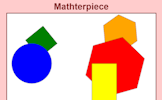#### Mathterpiece

Memorise a picture made up of geometrical shapes

### 8th Lesson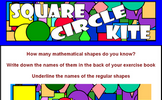#### Square Circle Kite

Write down the names of all the mathematical shapes you know.

### 9th Lesson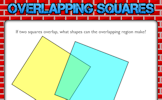#### Overlapping Squares

If two squares overlap, what shapes can the overlapping region make?

Some of the Starters above are to reinforce concepts learnt, others are to introduce new ideas while others are on unrelated topics designed for retrieval practice or and opportunity to develop problem-solving skills.

White Rose ResourcesEnd of block assessments provide a quick progress check at the end of each block of learning to make sure students have understood the content covered. This Scheme of Learning was produced by White Rose Maths and is used here with permission granted on 30th June 2021.For All: